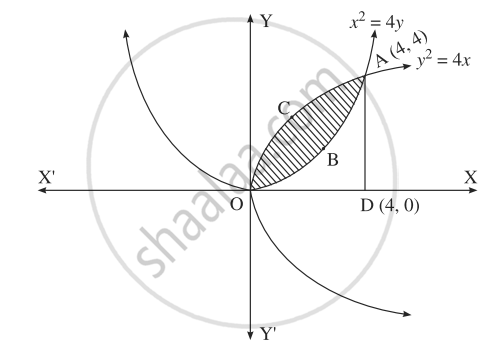# Solve the following : Find the area of the region lying between the parabolas :y2 = 4x and x2 = 4y - Mathematics and Statistics

Sum

Solve the following :

Find the area of the region lying between the parabolas :
y2 = 4x and x2 = 4y

#### SolutionFor finding the points of intersection of the two parabolas, we equate the values of y2 from their equations.
From the equation x2 = 4y, y = x^2/(4)

∴ y = x^4/(16)

∴ x^4/(16) = 4x

∴ x4 – 64x = 0
∴ x(x3 – 64) = 0
∴ x = 0 or x3 = 64
i.e. x = 0 or x = 4
When x = 0, y = 0

When x = 4, y = (4^2)/(4) = 4

∴ the points of intersection are O(0, 0) and A(4, 4).
Required area = area of the region OBACO
= [area of the region ODACO] –  [area of the region ODABO]
Now, area of the region ODACO
= area under the parabola y2 = 4x,
i.e. y = 2sqrt(x) between x = 0 and x = 4

= int_0^4 2sqrt(x)*dx

= [2  (x^(3/2))/(3/2)]_0^4

= 2 xx (2)/(3) xx 4^(3/2) - 0

= (4)/(3) xx (2^3)

= (32)/(3)
Area ofthe region ODABO
= area under the rabola x2 = 4y,
i.e. y = x^2/(4) between x = 0 and x = 4

= int_0^4 (1)/(4)x^2*dx

= (1)/(4)[x^3/(3)]_0^4

= (1)/(4)(64/3 - 0)

= (16)/(3)

∴ required area = (32)/(3) - (16)/(3)

= (16)/(3)"sq units".

Concept: Area Bounded by the Curve, Axis and Line
Is there an error in this question or solution?
Chapter 5: Application of Definite Integration - Miscellaneous Exercise 5 [Page 190]

#### APPEARS IN

Balbharati Mathematics and Statistics 2 (Arts and Science) 12th Standard HSC Maharashtra State Board
Chapter 5 Application of Definite Integration
Miscellaneous Exercise 5 | Q 2.04 | Page 190
Share